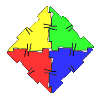#### You may also like### Polydron

This activity investigates how you might make squares and pentominoes from Polydron.### Eight Dominoes

Using the 8 dominoes make a square where each of the columns and rows adds up to 8### Prime Magic

Place the numbers 1, 2, 3,..., 9 one on each square of a 3 by 3 grid so that all the rows and columns add up to a prime number. How many different solutions can you find?

# Symmetry Challenge

##### Age 7 to 11 Challenge Level:

The $3$ by $3$ grid has $4$ lines of symmetry.
How might this help?
Flips or Turns / Reflections-- are they the same or different?
Try shading $1$ square only. What symmetries are possible?
Try shading $2, 3, 4 \ldots$ squares only. What symmetries are now possible?

How helpful would this table be?

 Lines of symmetry 0 1 2 3 4 Shaded Squares 1 2 3 4

What omissions do you notice?